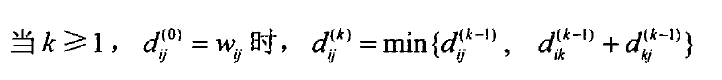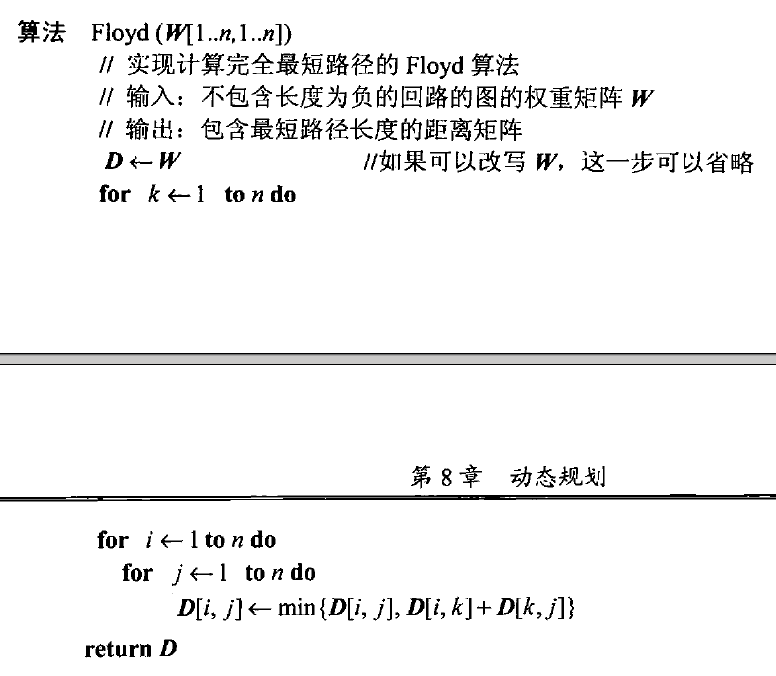# 算法笔记-动态规划

Posted by MaggicQ on June 18, 2017

# 动态规划

## 三个基本例子

• 币值最大化问题：给定一排硬币，其面值均为正整数$c_1, c_2, \cdots , c_n$ ，这些整数并不一定两两不同。请问如何选择硬币，使得在其原始位置互不相邻的条件下，所选硬币的总金额最大。

设最大可选金额为$F(n)$ ，那么：

$F(n) = max{c_n + F(n-2), F(n-1)} , n > 1$

且$F(0) = 0 , F(1) = c_1$

那么通过从左到右计算一行表格，就能够求出$F(n)$

需要耗费$\Theta(n)$时间和$\Theta(n)$ 空间。

• 找零问题：需找零金额为$n$ ，最少要用多少面值为$d_1 < d_2 < \cdots < d_m$的硬币？

设$F(n)$为总金额为$n$的数量最少的硬币的数目，定义$F(0) = 0$

递推公式：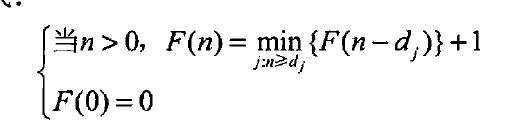伪代码：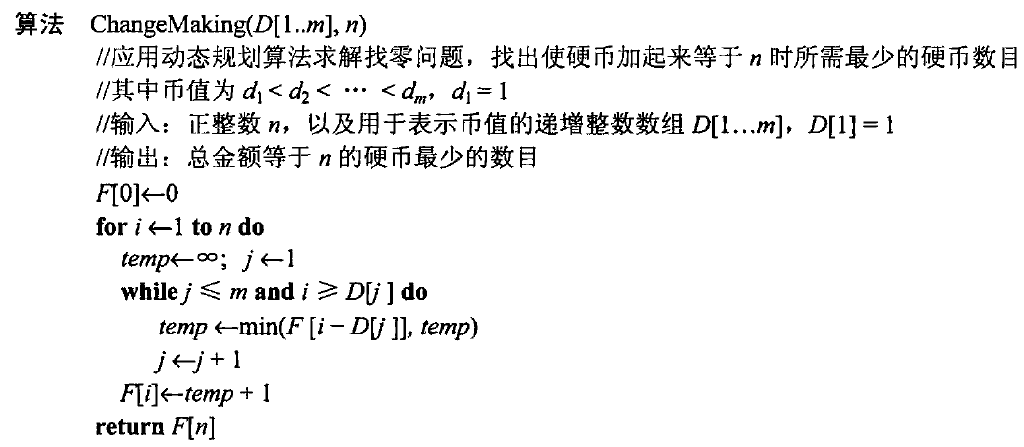• 硬币收集问题：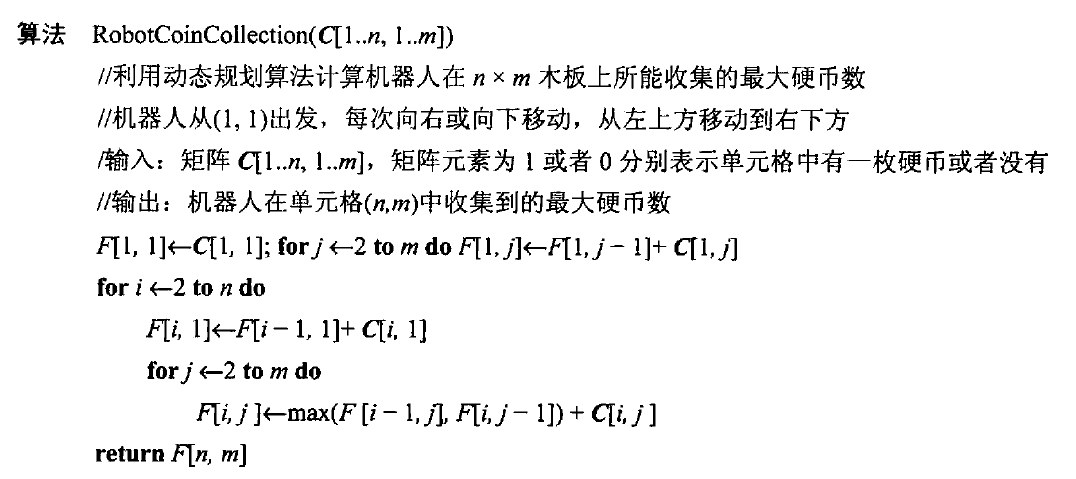## 背包问题和记忆功能

$F(i,j)$为该实例的最优解的物品总价值。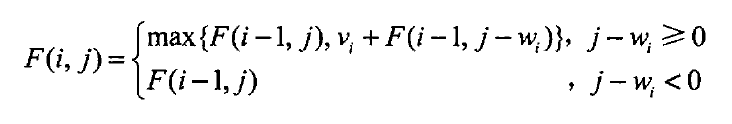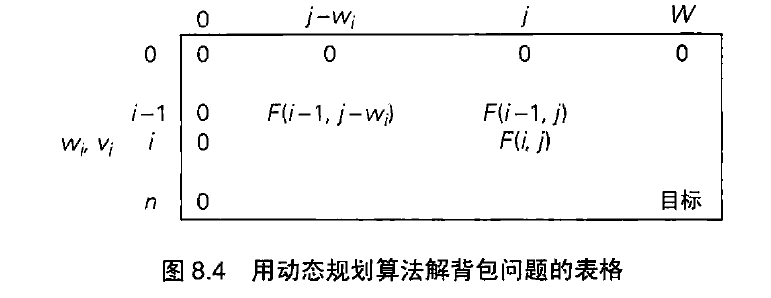时时间效率，空间效率都属于 $\Theta(nW)$

### 记忆化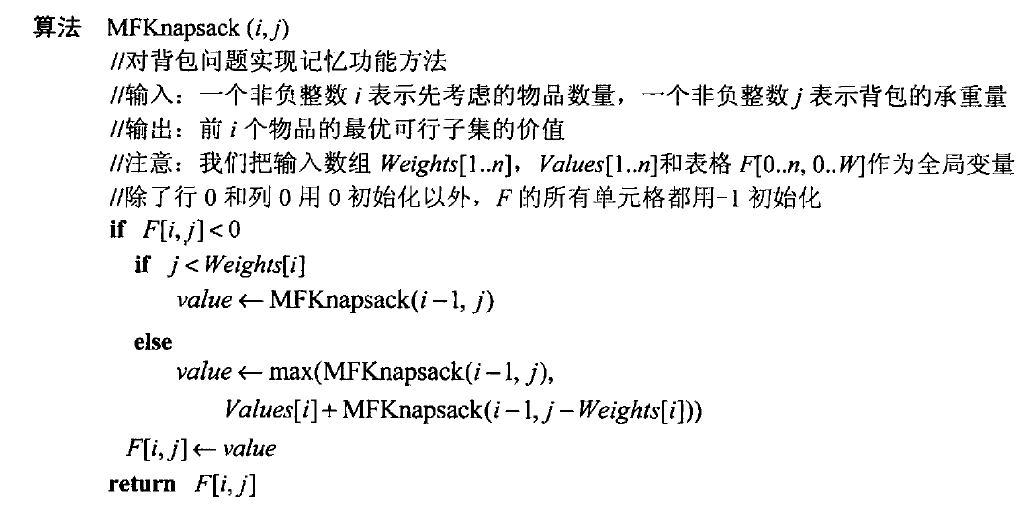## Warshall算法和Floyd算法

### Warshall算法

1，否则为0

$R^{(0)}, \cdots, R^{(k-1)}, R^{(k)} , R^{(n)}$

$j$个顶点之间存在一条有向路径，且路径的每一个中间顶点的编号不大于$k$时，

$r_{ij}^{(k)} = 1$

$r_{ij}^{k} = r_{ij}^{k-1} OR (r_{ik}^{(k-1)} AND r_{kj}^{(k-1)})$### 计算完全最短路径的Floyd算法

$D^{(0)} , \cdots, D^{(k-1)}, D^{(k)}, \cdots. D^{(n)}$